﻿ On Selection of Best Sensitive Logistic Estimator in the Presence of Collinearity

On Selection of Best Sensitive Logistic Estimator in the Presence of Collinearity

C. E. ONWUKWE, I. A. AKIOPEN ACCESSPEER-REVIEWED

On Selection of Best Sensitive Logistic Estimator in the Presence of Collinearity

C. E. ONWUKWE1,, I. A. AKI1

1Department of Mathematics/Statistics and Computer Science University of Calabar P. M. B. 1115, Cross River State, Nigeria

Abstract

Collinearity is a major problem in regression modeling. It affects the prediction ability of ordinary least square estimators. Collinearity is established in logistic regression models when the difference between the least and highest eigen value of the information matrix is more in relation to the least eigen value. This results in inflated variance of estimated regression parameters. Consequently, the resulting model is not reliable and will result in incorrect conclusions about the relationship among the variables. To overcome the problem of collinearity in logistic regression model a number of estimators were proposed. This article compares the performance of four estimators - ordinary logistic estimator, logistic ridge estimator, generalized logistic ridge estimator and modified logistic ridge estimator in the presence of collinearity, to ascertain which is more effective in variance reduction. To establish superiority among the above estimators, analysis is carried out on a case study in University of Calabar Teaching Hospital, Calabar Cross River State, Nigeria. Result showed that modified logistic estimator performed better than other estimator considered due to the fact that it had the smallest variance.

• ONWUKWE, C. E., and I. A. AKI. "On Selection of Best Sensitive Logistic Estimator in the Presence of Collinearity." American Journal of Applied Mathematics and Statistics 3.1 (2015): 7-11.
• ONWUKWE, C. E. , & AKI, I. A. (2015). On Selection of Best Sensitive Logistic Estimator in the Presence of Collinearity. American Journal of Applied Mathematics and Statistics, 3(1), 7-11.
• ONWUKWE, C. E., and I. A. AKI. "On Selection of Best Sensitive Logistic Estimator in the Presence of Collinearity." American Journal of Applied Mathematics and Statistics 3, no. 1 (2015): 7-11.

 Import into BibTeX Import into EndNote Import into RefMan Import into RefWorks

1. Introduction

Ordinary Least Squares (OLS) estimation is widely used in regression analysis. Logistic regression has proven to be one of the most versatile techniques in generalized linear models which allows for the modeling of categorical variables. Method of least squares performs well under some basic assumption such as where error are independent and following normal distribution with mean zero and having constant variance (Jadhav and Kashid, 2011). In real life situation, some variables are seen to relate with each other thereby introducing multicollinearity in models.

Presence of multicollinearity can make ordinary least square estimator to be unstable due to large variances which lead to poor prediction (Batah et al, 2008; Batah, 2011; Joshi, 2012; Nja, 2013). To overcome this problem, several measures had been presented. Remedies include ridge regression method by Hoerl and Kennard, (1970) and the iterative principal component method Marx and Smith (1990). Since multicollinearity produces large variances in ordinary least square estimation, ridge regression attempts to find parameter estimates that have smaller variance and hence smaller MSE by enlarging the small Eigen values (Nelder and Wedderburn, 1972; Hawkin and Yin, 2002; Vago and Kemeny, 2006).

2. Ordinary Ridge Regression Estimator

Consider a multiple linear regression model.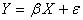(1)

Where Y is (nx1) vector of observations, β is a (px1) vector of unknown regression coefficients, X is a matrix of order (nxp) of observations on p predictor (regressor) variables x1, x2,…xp and e is an (nx1) vector of errors with E(e) = o and var(e) = σ2.

The least square estimator of β is given by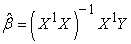.

The linear model can be written in canonical form as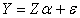(2)

where Z = XT, T is the matrix of eigen vectors of X'X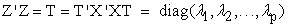where λi is the ith eigen value of X'X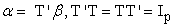The OLS estimator of α is given by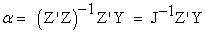(3)

where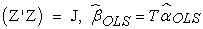(4)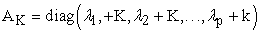(5)

where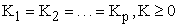K is a biasing constant.

K can be generalized as k = (K1, K2,…kp) so that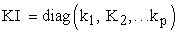The generalize ordinary Ridge estimator is obtained as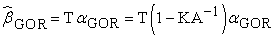(6)

where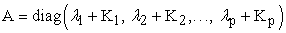λi is the ith eigen value of (X'X + KI)

This procedure is extended to model logistic ridge estimator and its subsequent modification, the modified logistic ridge regression estimator.

3. Ordinary Logistic Regression Estimator

The ordinary logistic estimator uses the iterative weighted least squares method. The ordinary logistic estimate of β is given by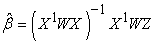(7)

where,

W is a diagonal matrix of weights

Z is a column matrix of adjusted dependent variables.

4. Logistic Ridge Regression Estimator

The generalized ridge regression can be expressed in canonical form as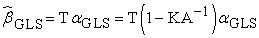(8)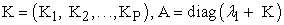λi is the ith eigen value of (X'WX + KI)

The logistic Ridge regression estimator of β is given by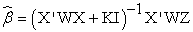(9)

5. Modified Logistic Ridge Regression Estimator

Modified logistic ridge regression estimator was proposed by Nja et al (2013). This is given in canonical form as follows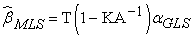(10)

Where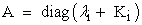λi is the ith eigen value of (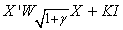) 0≤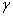≤1.

The modified logistic ridge estimator of β is given by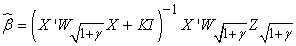(11)

6. Methodology

If the probability of an event taking place is P, then the odd of that event is given by: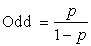That is, odd is the probability of an event taking place divided by the probability of the event not taking place. The log of the odds is known as logit given as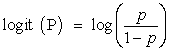Logistic regression like other regression has a dependent variable and independent variable(s). In logistic regression the dependent variable is a logit which is the natural log of the odds,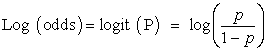Logistic regression is a modeling strategy that relates the logit to a set of explanatory variable with a linear model (Bender and Groven, 1997; Hosmer and Lemeshow, 2008; Lamote 2012). That is,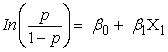where:

β0 = the constant

β1 = the regression coefficient

X = the predictor variable

So that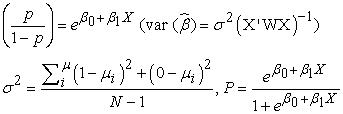7. The Model

We are modeling the probability that a person selected from a subpopulation has respiratory infection given by,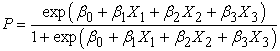where,

β0 = constant

β1 = sex

β2 = location

β3 = % of exposure

The estimation of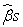are as follows:

i. Ordinary logistic estimator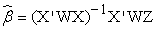W is a diagonal matrix of weights given by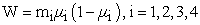where:

mi is the sub population total

µi is the response probability

and Z is a column matrix of adjusted dependent variate given by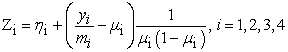where:

yi is number of favourable outcome

ii. Logistic Ridge Estimator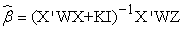Computation for Z and W are the same as those of ordinary logistic estimator.

KI is diagonal matrix of Tikhonov constants (small positive biasing constants).

where: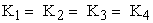iii. Generalized Logistic Ridge Estimator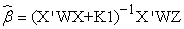The computation for Z, W and K are the same as those of logistic ridge except that;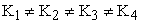iv. Modified Logistic Ridge Estimator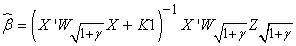where,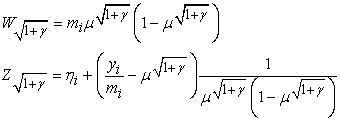The variance of the parameter is given by Var(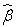) = σ2(X’WX)-1

where: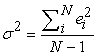where ei is the error.

The estimation of parameters and calculation of variances were done with MATLAB iteratively.

8. Data Collection

The data for this research were obtained from the University of Calabar Teaching Hospital, Calabar, in Cross River State of Nigeria. This was facilitated by a well structured questionnaire that was administered to patients attending the family medicine clinic of the hospital within a period of two weeks. A total of 180 questionnaires were issued out and 169 were properly filled and returned which is presented in Table 1. Data are obtained on location of patients’ residents, sex and levels of exposure. The explanatory variables are sex, location and percentage level of exposure of which the first two are dichotomous and the third is continuous. The response variable is dichotomous.

10. Fitted Model

The probabilities that a person selected from a sub group has respiratory infection as given by the different estimators are as follows:

1. Ordinary logistic estimator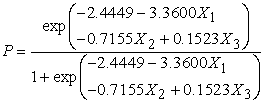2. Logistic ridge estimator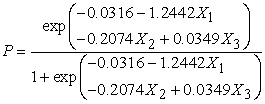3. Generalized logistic ridge estimator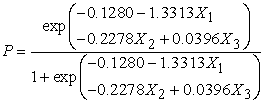4. Modified logistic ridge estimator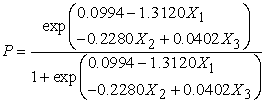From Table 3 (variances of the different estimators) we can see that modified logistic ridge estimator has the least variances of the parameters and hence we take the model obtained using modified logistic estimator.

The model given by modified logistic ridge estimator can be explained as follows:

1. The probability that a female living in a rural area with 20% level of exposure is 0.7116

2. The probability that a male living in a rural area with 26% level of exposure is 0.7144.

3. The probability that a female living in an urban centre with 39% level of exposure is 0.5879.

4. The probability that a male living in an urban centre with 42% level of exposure is 0.5616.

11. Discussion of Findings

Result presented in Table 2 show significant difference in the parameter estimates by the different estimators. It is observed that the estimates obtained by using ordinary logistic estimator is significantly different from those of the ridge estimators. In Table 3 it is seen that there is significant difference in the variances of the parameter estimates from the different estimators. Looking closely at the result, modified logistic ridge estimator is more sensitive and performs better than the other estimator due to its ability to reduce the variance associated with multicollinearity. The probability shows that males living in rural area with an exposure level of 39% have a higher probability of having respiratory infection.

12. Conclusion

Base on the findings of this study, it can be concluded that modified logistic ridge estimator is more superior to other estimators (ordinary logistic, logistic ridge and generalized logistic ridge) on the basis of variances of the parameter estimates. Also persons living in rural areas are seen to be more prone to having respiratory infection.

Acknowledgement

I acknowledge the efforts of Dr. M. E. Nja who has contributed immensely to the success of this work by putting me through the computational procedures involve. I also appreciate the effort of Mr. Kayode which has led to the actualization of the goal of this work.

References

  Batah, F. S. M. Ramanathan, T. V., Gore, S. D. (2008). The Efficiency of modified Jackknife and Ridge Type Regression Estimators: A comparison Surveys in Mathematics and its application 3 111-122.In article  Batah, F. S. (2011). A new Estimator by generalized Modified Jackknife Regression. Estimator: Journal of Basarah Researches (Sciences), 37 (4) 138-149.In article  Bender, R. and Grooven, U. (1997). Ordinal Logistic Regression in Medical Research. Journal of the Royal College of Physician of London. Sept/Oct 1997: v 31 (5): 546-551.In article PubMed  Hawkins, D. M. Yin, X. (2002). A faster algorithm for ridge regression. Computational statistics and data analysis. 40, 253-262.In article CrossRef  Hoerl, A. E. and Kennard, R. W. (1970). Ridge Regression Biased Estimation for non-Orthogonal Problems. Communication is statistics: Theory and Methods 4 105-123.In article  Hosmer, D. w. and Lemeshow, S. (2008). Applied Logistic Regression 2nd Edition. Wiley.In article  Joshi, H. (2012). Multicollinearity Diagnosis in Statistical Modeling and remedies to deal with it using bars. Cytel Statistical Software Services PVT Ltd. Pone India.In article  Judhav, N. H. & Kashid, D. N. (2011). A jackknife Ridge M. Estimator for Regression models with multicollinearity and outliers. Journal of statistical theory and practice. 5: 4, 659-673.In article  Lamote, W. W. (2012). Multiple Logistic Regression. Boston. Boston University Press.In article  Marx, B. D. and Smith, E. P. (1990). Principal component estimation for generalized regression. Biometrika. 77 (1): 23-31 (1990).In article CrossRef  Nelder, J., Wedderburn, R. W M. (1972). Generalized Linear Models. Journal of the Royal Statistical society, A 135, 370-384.In article CrossRef  Nja, M. E. (2013). A new Estimation procedure for Generalized Linear Regression Designs with near Dependencies. Accepted for publication. Journal of Statistical; Econometric Methods.In article  Nja, M. E., Ogoke, U. P. & Nduka, E. C. (2013). The logistic Regression model with a modified weight function. Journal of statistical and econometric Method, Vol. 2 No. 4 2013. 161-171.In article  Vago, E. & Kemeny, S. (2006). Logistic Ridge Regression for clinical Data Analysis (A case study). Applied Ecology and Environmental Research 4 (2) 171-179.In article CrossRef